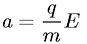Equations > Physics > Electricity and Magnetism > Acceleration of a particle in an electric field

### Acceleration of a particle in an electric fieldLatex Code:

MathML Code:

 $a=\fracqmE$

MathType 5.0: